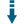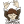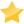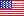# Equation Operating System: Solve equations with multiple variablesDownload .zip
 InfoExample DemosView files (17)Download .zip Reputation Support forum Blog Links
 Ratings Unique User Downloads Download Rankings61% Total: 2,009 All time: 1,943 This week: 258Version License PHP version Categories eos 3.2.1 GNU Lesser Genera... 5.3 Graphics, Math
Description Author

This class solves equations with multiple variables.

It does not resort to the PHP eval() function to help prevent security risks of using malicious expression values for instance with a calculator that takes expressions defined by the users.

It comes with a sub-class that can produce graphic charts plotting the curve defined by the equation expression withing a given range of x and y values. The generate chart graphics can be outputted in the PNG or JPEG formats using the GD library.

Innovation AwardJanuary 2005 Number 2Prize: One downloadable copy of Komodo Pro Solving equations can have many applications, like for instance, drawing charts that represent the curves defined by assigning values of given ranges to the variables of the equations. The PHP eval() function can be used to dynamically evaluate the expressions defined by an equation. However, it may be dangerous to evaluate an arbitrary expression provided by an user, as it may contain malicious code. This class provides an alternative solution that consists in a full expressions parser and evaluator that only supports a limited set of operations that do not represent a security risk when the operations are evaluated. Manuel LemosPerformance Level
Name: Classes: Jon Lawrence `` 4 packages by Jon LawrenceUnited States 37 790 101 in United States58368 in United StatesLevel 1Innovation awardNominee: 1x

Details

# EOS

## Installation

Install EOS with Composer

``````"require": {
"jlawrence/eos": "3.*"
}
``````

Run `composer update` and you're done.

## Equation Operating System

### jlawrence\eos\

This class makes it incredibly easy to use and parse/solve equations in your own applications. __NOTE__ ALL of the functions within these classes are static. It is also important to note that these classes throw exceptions if running in to errors, please read the beginning of the `Math.php` file for the defines of the exceptions thrown. Exceptions includes a descriptive message of the error encountered and within `Parser` will also typically include the full equation used.

#### Parser

This class has one important function, `Parser::solve()` which does all the legwork, so we'll start there and end with examples.

``````use jlawrence\eos\Parser;
``````

##### solve(\$infix, \$variables)

To use this function:

``````\$value = Parser::solve(\$eq, \$vars);
``````

###### _\$infix_

Is simply a standard equation with variable support.

Example Equations:

``````2(4x)
5+((1+2)*4)+3
5+4(1+2)+3
10*sin(x)
10*cos(x)
``````

The parser has good implied multiplication.

###### _\$variables_

The variables are fairly simple to understand. If it contains a scalar (ie a non-array value) _every_ variable within the equation will be replaced with that number. If it contains an array, there will be a by-variable replacement - note that the array MUST be in the format of `'variable' => value` Such as:

``````array(
'x' => 2,
'y' => 3
);
``````

Given the equation:

``````5x^y
``````

If this is called by:

``````Parser::solveIF('5x^y', 2);
``````

It will equal '20', as every variable is replaced by 2. However, if called like:

``````Parser::solveIF('5x^y', array(
'x' => 2,
'y' => 3));
``````

You will get the result of '40' as it would equate to `5*2^3`, as expected.

#### jlawrence\eos\Graph

To use:

``````use jlawrence\eos\Graph;
``````

This is the fun class that can create graphs. The image will default to 640x480, to initialize a different size use:

``````Graph::init(\$width, \$height);
``````

The `\$width` and `\$height` are the values used for the image size.

##### graph(\$eq, \$xLow, \$xHigh, [\$xStep, \$xyGrid, \$yGuess, ...])

This method will generate the graph for the equation (`\$eq`) with a min and max `x` range that it will parse through. All Variables explained:

• `\$eq` The Standard Equation to use. _Must_ have a variable in it. (ie `x`)
• `\$xLow` The starting point for the calculations - the left side of the graph.
• `\$xHigh` The last point calculated for the variable - the right side of the graph.
• `\$xStep` Stepping point for the variable. Set to null/false to use the smart xStep feature within the graph class.
• `\$xyGrid = false` Show `x/y` gridlines on the graph. Defaults to false. Each grid line is set at an integer, with a max of 30 lines, so it will calculate the stepping for it. When the grid is show, the lines are labeled along the top and left side of the image.
• `\$yGuess = true` Guess the Lower and Upper `y-bounds` (The bottom and top of the image respectively.) This will set the the bounds to the lowest `y` value encountered for the `\$yLow`, and the largest `y` value for `\$yHigh`.
• `\$yLow = null` Lower bound for `y`. Will be reset if a lower value for `y` is found if `\$yGuess` is true.
• `\$yHigh = null` Upper bound for `y`. Will be reset if a larger `y` value is found if `\$yGuess` is true.

If you don't want the axis' labeled with their numbers, you can turn off the default behavior with:

``````Graph::\$labelAxis = false;
``````

TODO:

• Allow user-defined colors for all aspects of the graph.

To set up a graph with a `21x21` window (ie `-10 to 10`) for the equation `sin(x)` and output as PNG, would use as:

``````Graph::graph('sin(x)', -10, 10, 0.01, true, false, -10, 10);
Graph::outPNG();
``````

It would look like:## Development

### Testing

Run the unit tests by first installing phpunit with (from the repository root)

``````composer update
``````

Then run the tests with

``````phpunit
``````

When creating classes for adding functions to the package, make sure to call `Parser::solveIF()` instead of `Parser::solve()` so that the class retains the full original equation used by the user.Files
File Role Descriptionsrc (7 files)tests (1 directory)README.md Doc. Description of class functionality and use cases.codeclimate.yml Data For testing.travis.yml Data For testingcomposer.json Data Auxiliary dataindex.php Example Example usage of graphing functionphpunit.xml Data For testingFiles / src
File Role DescriptionAdvancedFunctions.php Class Class sourceGraph.php Class Class sourceMath.php Class Class sourceMatrix.php Class Class sourceParser.php Class Class sourceStack.php Class Class sourceTrig.php Class Class sourceFiles / tests
File Role Descriptionunit (4 files)Files / tests / unit
File Role DescriptionGraphTest.php Test For testingMatrixTest.php Test For testingParserTest.php Test Unit test scriptStackTest.php Test For testing

 All time: 1,943 This week: 258All time Utility: 95%85%- 55%- - 61%1213Advertise on this siteSite mapNewsletterStatisticsSite tipsPrivacy policyContact
For more information send a message to `info at phpclasses dot org`.